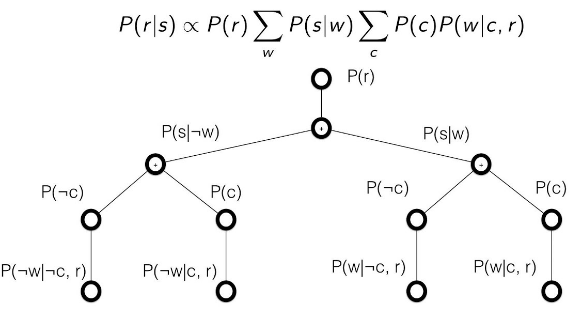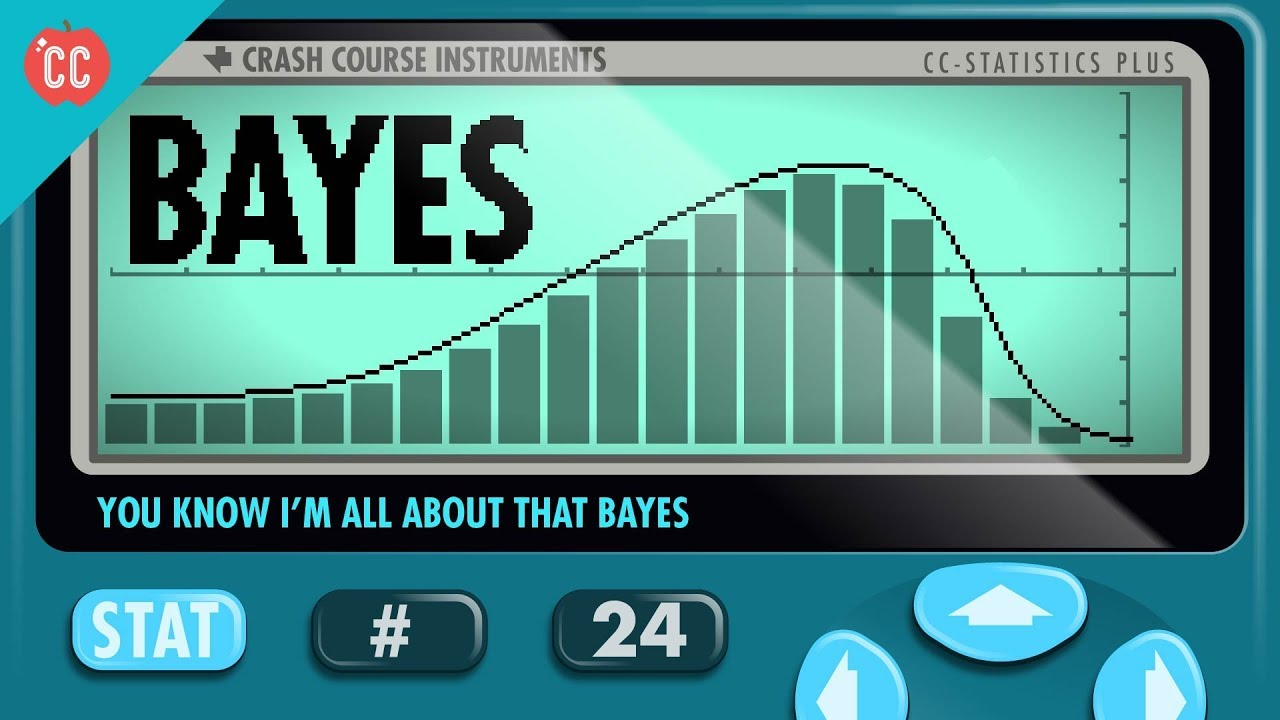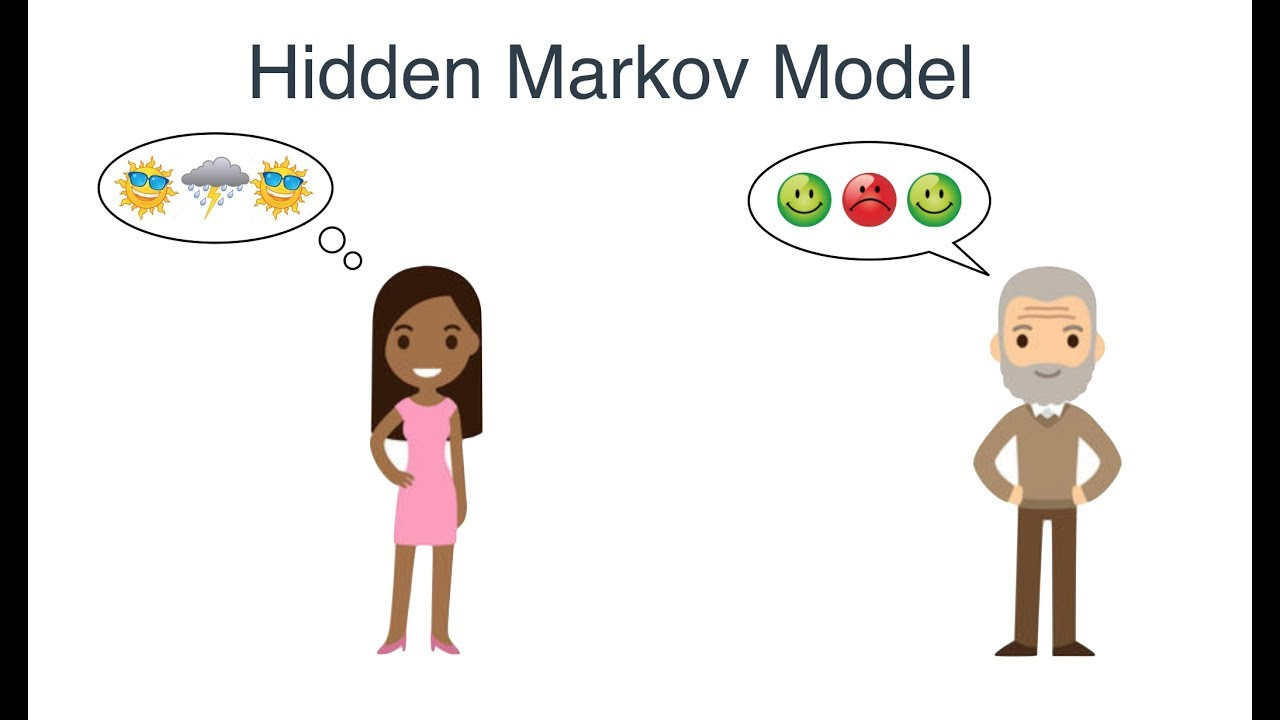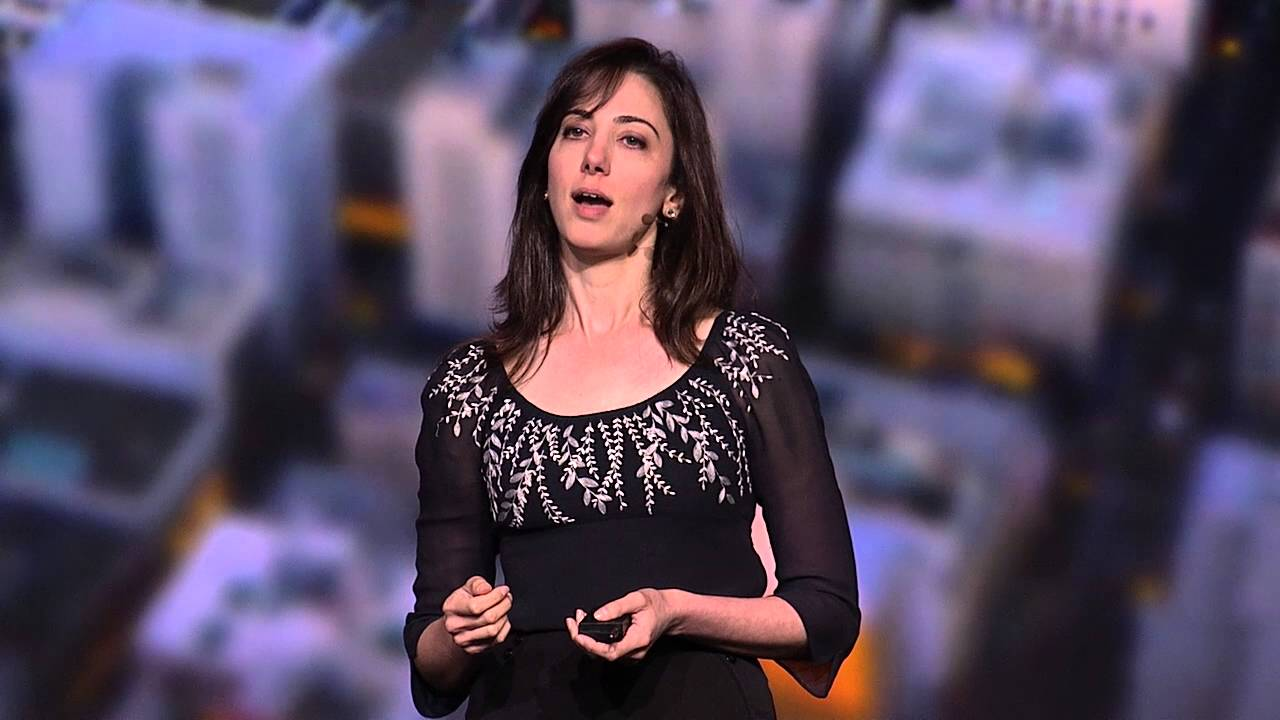# BayesStatistics

#### Is Bayesian thinking a sham?

Here’s a thought provoking video by Julia Galef  There’s a common criticism that people who claim to practice “Bayesian thinking” aren’t actually doing anything special — they’re just using a fancy term to make their opinions seem more objective than they really are. In this video I explain why that criticism is misguided.Mathematics Statistics

#### Bayes Theorem and Making Probability Intuitive

3Blue1Brown explores perhaps the most important formula in probability: Bayes Theorem. The study with Steve: https://science.sciencemag.org/content/185/4157/1124 http://www.its.caltech.edu/~camerer/Ec101/JudgementUncertainty.pdfDeep Learning Quantum Computing

#### Bayesian Deep Learning on a Quantum Computer

Learn about bayesian deep learning and how to apply it to a quantum computer from Alex Pozas-KerstjensMathematics Statistics

#### Bayesian Statistics with Hannah Fry

You’ll hear the term Bayes or Bayesian come up a lot in data science, but this video explores the theory with tennis balls and a table.Data Science Mathematics

#### 15 Great Articles about Bayesian Methods and Networks

Here’s a curated list of articles on Bayesian methods and networks. An Introduction to Bayesian Reasoning Basics of Bayesian Decision Theory How Bayesian Inference Works Marketing Insight from Unsupervised Bayesian Belief Networks Bayesian Nonparametric Models Using Bayesian Kalman Filter to predict positions of moving particles Naive Bayes Classification explained with Python code Wheel Of Fortune […]Machine Learning Mathematics

#### Bayes’ Theorem Problems, Definition & Examples

Here’s a great exploration of Bayes’ Theorem and how to use it in real world problems. Bayes’ theorem is a way to figure out conditional probability. Conditional probability is the probability of an event happening, given that it has some relationship to one or more other events. For example, your probability of getting a parking […]Data Science

In case my previous post had left you wanting to know more about Bayes’ Theorem. With apologies to Meghan Trainor.AI Data Science

#### A Friendly Introduction to Bayes Theorem and Hidden Markov Models

Here’s a great introduction to Bayes Theorem and Hidden Markov Models, with simple examples. If you understand basic probability, then you can follow along.Machine Learning

#### How Bayes Theorem works

The great Brandon Rohrer explains how Bayes’ Theorem works in this video. For another look at Bayesian thinking, check out Julia Galef and some of the posts I’ve written featuring her videos.